﻿ How do I? > Merge datasets

# Merge datasets

Easy Data Transform has two options for merging two datasets. Stack and Join.

## Stack datasets

If you want to merge the two datasets so they are one on top of another, use the Stack transform. For example, to Stack these two datasets: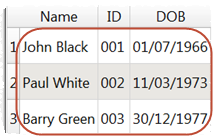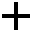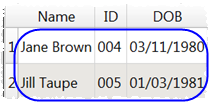To get this dataset:Drag the two dataset files onto the Center pane of Easy Data Transform.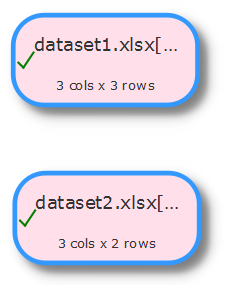Select the two datasets using Ctrl+click then click the Stack transform in the Left pane.The datasets are now stacked in the vertical order that the datasets are shown on the screen. The top dataset is shown first. You can swap the the vertical positions of the datasets to change the order in which they are stacked.

If you want to stack column n of the first dataset above column n of the second dataset, set Align columns by to Column number.

If you want to stack columns by common header names (even if they aren't in the same order), set Align columns by to Header name.

If you want to stack a large number of files you can do it by using batch processing to write to an output item with Write Mode=Append.

## Join datasets

If you want to merge the two datasets side-by-side using a common ('key') column, use the Join transform. For example, to Join these two datasets:By common ID value to get this dataset:Drag the two dataset files onto the Center pane of Easy Data Transform.Select the two datasets using Ctrl+click then click the Join transform in the Left pane.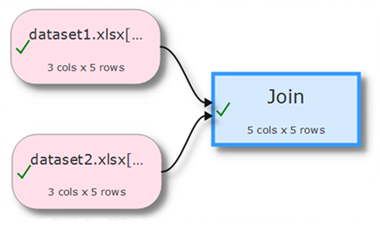Set both Top key column and Bottom key column to the common ('key') column.

The datasets are now joined side-by-side using the common column. The top dataset is shown on the left. You can swap the the vertical positions of the datasets to change the order in which they are joined.

If you just want to join row N of one dataset to row N of another dataset, you can use the Row Num transform to create a common column in each dataset.

Set Include top non-matching rows and Include bottom non-matching rows depending on what you want to do with top and bottom dataset rows for which there are no matches.

Note that matching columns takes account of whitespace and case. So you might need to do Trim and Case transforms before the join.